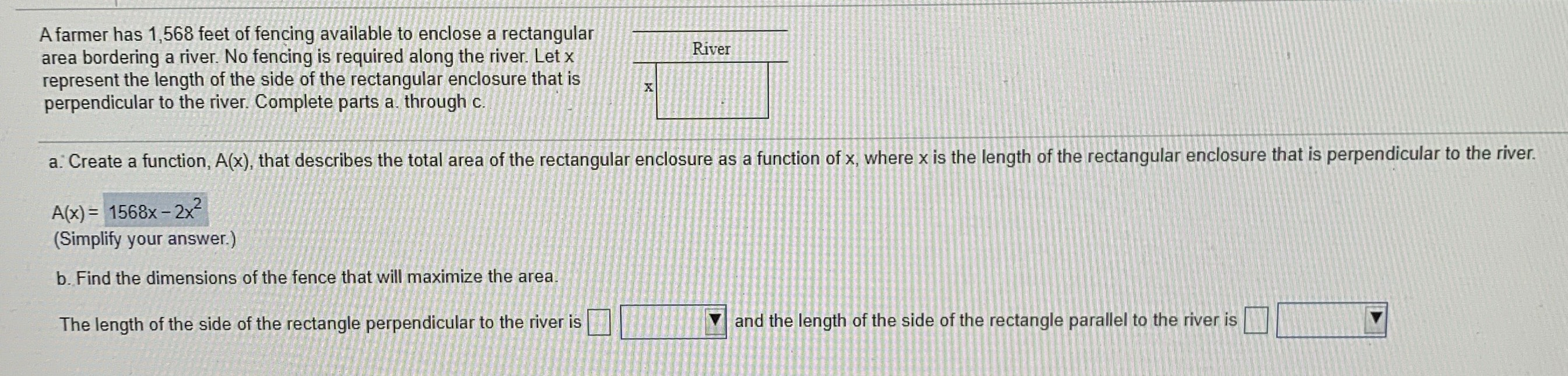### ¿Todavía tienes preguntas de matemáticas?

Pregunte a nuestros tutores expertos
Algebra
PreguntaA farmer has $$1,568$$ feet of fencing available to enclose a rectangular area bordering a river. No fencing is required along the river. Let $$x$$ represent the length of the side of the rectangular enclosure that is perpendicular to the river. Complete parts a through c.

a. Create a function, $$A ( x )$$ , that describes the total area of the rectangular enclosure as a function of $$x$$ , where $$x$$ is the length of the rectangular enclosure that is perpendicular to the river. A(X)=______?

b. Find the dimensions of the fence that will maximize the area. The length of the side of the rectangle perpendicular to the river is _____and the length of the side of the rectangle parallel to the river is _____